# Ceil Node

Access: NodesMathCeil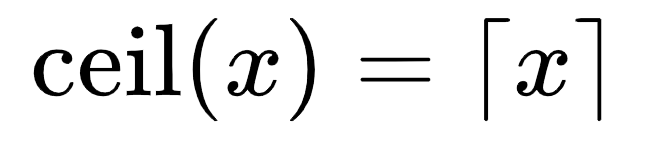The Ceil node rounds up values to the nearest whole number or integer. For example, 0.3 becomes 1, 1.7 becomes 2, 3.2 becomes 4.

Note:  The Ceil node is similar to the Floor node operation, except, instead of rounding down to the nearest whole number, Ceil rounds up. For more information, see the Floor node.

 Input Output 3.75, 5.08, 2.49, 1.0 4, 6, 3, 1 5.0, 6.5, 7.2, 1.0 5, 7, 8, 1 0.0, 0.3, 0.7, 0.0 0, 1, 1, 0

# Ceil Node Inputs

 Input The input values to be rounded up to the nearest integer.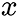# Ceil Node Outputs

 Output The input after rounding up to the nearest whole number.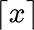# Workflow Example

The Ceil node can be used to create a simple posterize effect from a texture color input. To define the number of levels in the posterize effect, you can use the Multiply and Divide nodes, in the following way, where the Multiply and Divide B inputs are the number of levels you would like in the posterize effect: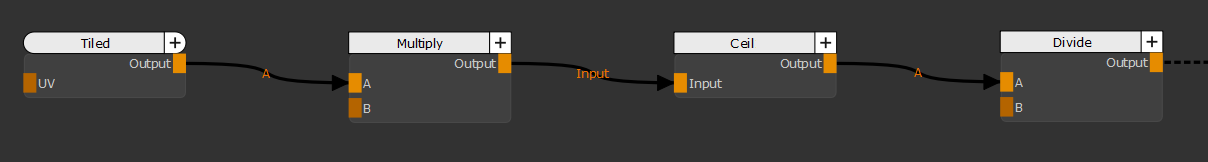Workflow to create a posterize effect using a Ceil node

Note:  The Ceil node produces a similar posterize effect to the Floor Node but because it rounds the input values up, the resulting colors will appear lifted.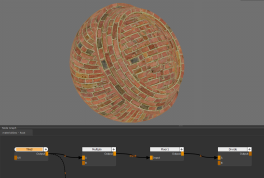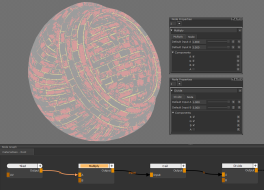Input Texture Multiply and Divide B input of 3. Resulting in 3 Posterize Levels.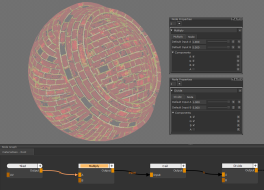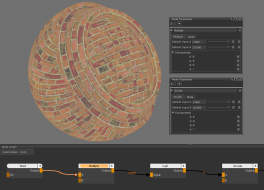Multiply and Divide B input of 5. Resulting in 5 Posterize Levels. Multiply and Divide B input of 10. Resulting in 10 Posterize Levels.